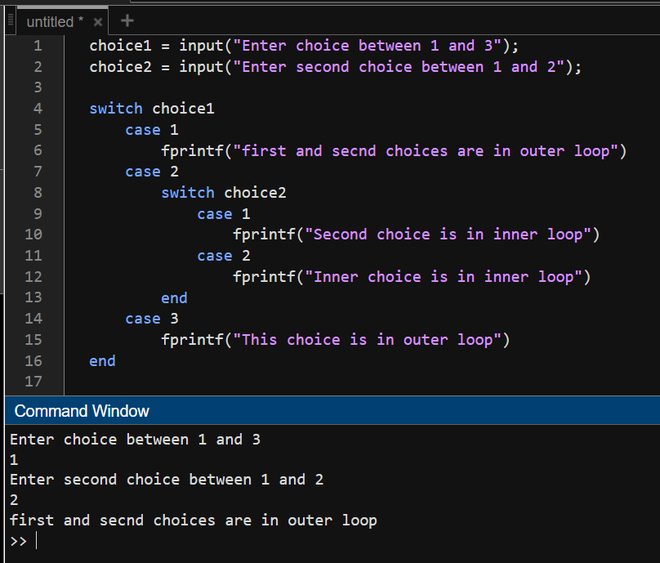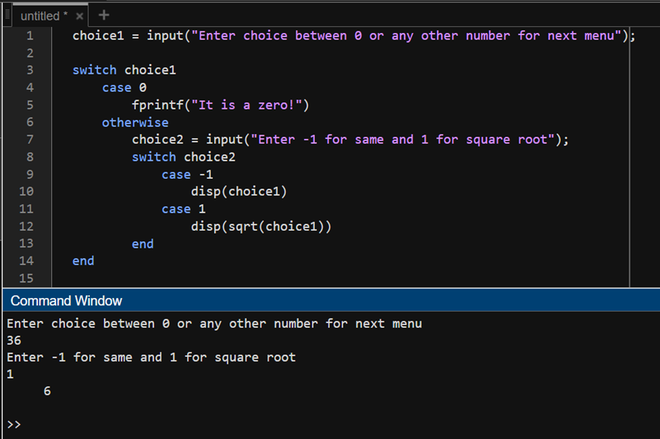Open in App
Not now

# Nested Switch in MATLAB

• Last Updated : 21 Nov, 2022

Switch statements in MATLAB allow having multiple cases as conditionals. It is an implementation of the simple if-elif-else-end cycle but, better. In this article, we will see how nested switch statements work in MATLAB with the help of example.

### Syntax:

switch choice_1

case <1>

switch choice_2

case <B>

statements

.

end    %end of inner switch

.

end   %end of outer switch

Now we see the below example printing the switch whether it is inner or outer.

Example 1:

## Matlab

 `% MATLAB Code for switch``choice1 = input(``"Enter choice between 1 and 3"``);``choice2 = input(``"Enter second choice between 1 and 2"``);` `switch` `choice1``    ``case` `1``        ``fprintf(``"first and second choices are in outer loop"``)``    ``case` `2``        ``switch` `choice2``            ``case` `1``                ``fprintf(``"Second choice is in inner loop"``)``            ``case` `2``                ``fprintf(``"Inner choice is in inner loop"``)``        ``end``    ``case` `3``        ``fprintf(``"This choice is in outer loop"``)``end`

Output:Now we will use the nested switch for the situation where if the input number is 0, it prints “It is zero!” else if it is not 0, it will ask for new choice whether you want to print the number or its square root.

Example 2:

## Matlab

 `% MATLAB Code for Nested Switch case``choice1 = input(``"Enter choice between 0 or any other number for next menu"``);` `switch` `choice1``    ``case` `0``        ``fprintf(``"It is a zero!"``)``    ``otherwise``        ``choice2 = input(``"Enter -1 for same and 1 for square root"``);``        ``switch` `choice2``            ``case` `-1``                ``disp(choice1)``            ``case` `1``                ``disp(sqrt(choice1))``        ``end``end`

Output:My Personal Notes arrow_drop_up